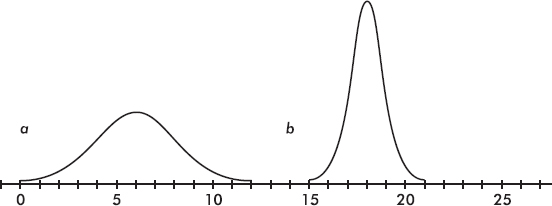# AP Statistics Multiple-Choice Practice Questions: The Normal Distribution 1

### Test Information8 questions18 minutes

1. Which of the following is a true statement?

2. Which of the following is a true statement?

3. Populations P1 and P2 are normally distributed and have identical means. However, the standard deviation of P1 is twice the standard deviation of P2. What can be said about the percentage of observations falling within two standard deviations of the mean for each population?

4. Consider the following two normal curves:Which has the larger mean and which has the larger standard deviation?

5. Assume the given distributions are normal. A trucking firm determines that its fleet of trucks averages a mean of 12.4 miles per gallon with a standard deviation of 1.2 miles per gallon on cross-country hauls. What is the probability that one of the trucks averages fewer than 10 miles per gallon?

6. Assume the given distributions are normal. A factory dumps an average of 2.43 tons of pollutants into a river every week. If the standard deviation is 0.88 tons, what is the probability that in a week more than 3 tons are dumped?

7. Assume the given distributions are normal. An electronic product takes an average of 3.4 hours to move through an assembly line. If the standard deviation is 0.5 hour, what is the probability that an item will take between 3 and 4 hours?

8. Assume the given distributions are normal. The mean score on a college placement exam is 500 with a standard deviation of 100. Ninety-five percent of the test takers score above what?